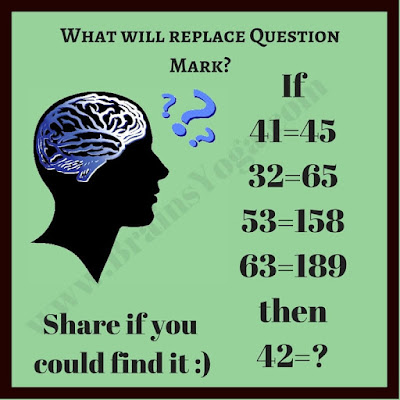This is Maths Logic Puzzle to test your logic and Maths skills. Solving this puzzle will help you to improve your mental ability. In this puzzle image, there are number equations. Find the hidden logic in these number equations and find the value of the missing number. Can you find what will replace the question mark?What will replace the question mark?

Unknown said...

86